### Home > MC1 > Chapter 9 > Lesson 9.2.2 > Problem9-71

9-71.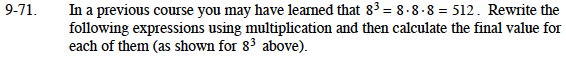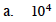Each exponent (the smaller number to the top right of the 10) represents another 10 by which to multiply.

(10)(10)(10)(10) = 10,000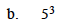What is the exponent in this problem?
That will be the number of fives to multiply together.

(5)(5)(5) = 125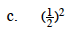Remember, when multiplying fractions, you simply multiplythe numerators together and denominators together.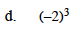The product of three negative numbers is a negative number.